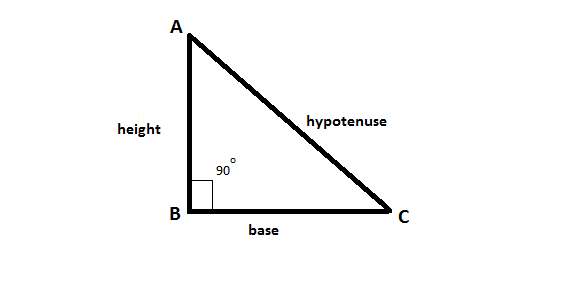# Find the height of a right-angled triangle whose area is X times its base

Given that the area of a right-angled triangle is X times its base b. The task is to find the height of the given triangle.Examples:

Input: X = 40
Output: 80

Input: X = 100
Output: 200

## Recommended: Please try your approach on {IDE} first, before moving on to the solution.

Approach: We know that the area of a right-angled triangle, Area = (base * height) / 2 and it is given that this area is X times the base i.e. base * X = (base * height) / 2.
Solving for height, we get height = (2 * base * X) / base = 2 * X.

Below is the implementation of the above approach:

## C++

 `// C++ implementation of the approach ` `#include ` `using` `namespace` `std; ` ` `  `// Function to return the height of the ` `// right-angled triangle whose area ` `// is X times its base ` `int` `getHeight(``int` `X) ` `{ ` `    ``return` `(2 * X); ` `} ` ` `  `// Driver code ` `int` `main() ` `{ ` `    ``int` `X = 35; ` ` `  `    ``cout << getHeight(X); ` ` `  `    ``return` `0; ` `} `

## Java

 `// Java implementation of the approach ` `import` `java.util.*; ` `import` `java.lang.*; ` `import` `java.io.*; ` ` `  `class` `Gfg ` `{ ` `     `  `// Function to return the height of the ` `// right-angled triangle whose area ` `// is X times its base ` `static` `int` `getHeight(``int` `X) ` `{ ` `    ``return` `(``2` `* X); ` `} ` ` `  `// Driver code ` `public` `static` `void` `main (String[] args) ``throws` `java.lang.Exception ` `{ ` `    ``int` `X = ``35``; ` `    ``System.out.println(getHeight(X)) ; ` `} ` `} ` ` `  `// This code is contributed by nidhiva `

## Python3

 `# Python 3 implementation of the approach ` ` `  `# Function to return the height of the ` `# right-angled triangle whose area ` `# is X times its base ` `def` `getHeight(X): ` `    ``return` `(``2` `*` `X) ` ` `  `# Driver code ` `if` `__name__ ``=``=` `'__main__'``: ` `    ``X ``=` `35` ` `  `    ``print``(getHeight(X)) ` ` `  `# This code is contributed by ` `# Surendra_Gangwar `

## C#

 `// C# implementation of the approach ` `using` `System; ` ` `  `class` `Gfg ` `{ ` `     `  `// Function to return the height of the ` `// right-angled triangle whose area ` `// is X times its base ` `static` `int` `getHeight(``int` `X) ` `{ ` `    ``return` `(2 * X); ` `} ` ` `  `// Driver code ` `public` `static` `void` `Main ()  ` `{ ` `    ``int` `X = 35; ` `    ``Console.WriteLine(getHeight(X)) ; ` `} ` `} ` ` `  `// This code is contributed by anuj_67.. `

Output:

```70
```

My Personal Notes arrow_drop_upCheck out this Author's contributed articles.

If you like GeeksforGeeks and would like to contribute, you can also write an article using contribute.geeksforgeeks.org or mail your article to contribute@geeksforgeeks.org. See your article appearing on the GeeksforGeeks main page and help other Geeks.

Please Improve this article if you find anything incorrect by clicking on the "Improve Article" button below.

Improved By : nidhiva, vt_m, SURENDRA_GANGWAR

Article Tags :
Practice Tags :

Be the First to upvote.

Please write to us at contribute@geeksforgeeks.org to report any issue with the above content.